﻿ 集合交集问题的安全计算：解读
﻿• 价格透明
• 信息保密
• 进度掌控
• 售后无忧# 集合交集问题的安全计算：解读

## 摘要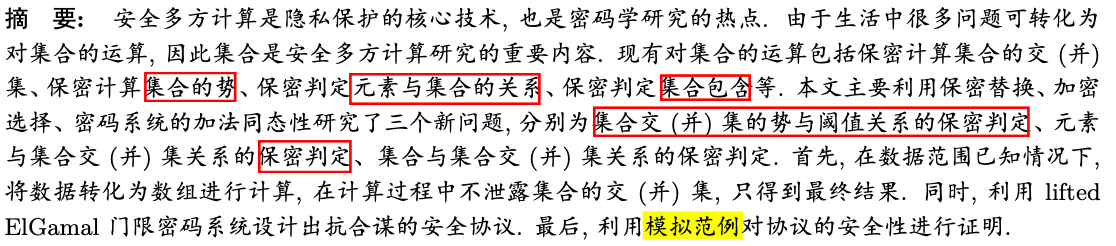（1）集合的势：交/并集个数
（2）该文主要讨论三点：

• 集合的势与阈值的大小关系
• 集合的交/并集与元素的包含关系
• 集合的交/并集的判定

（3）模拟范例：是安全性证明的方法

## 引言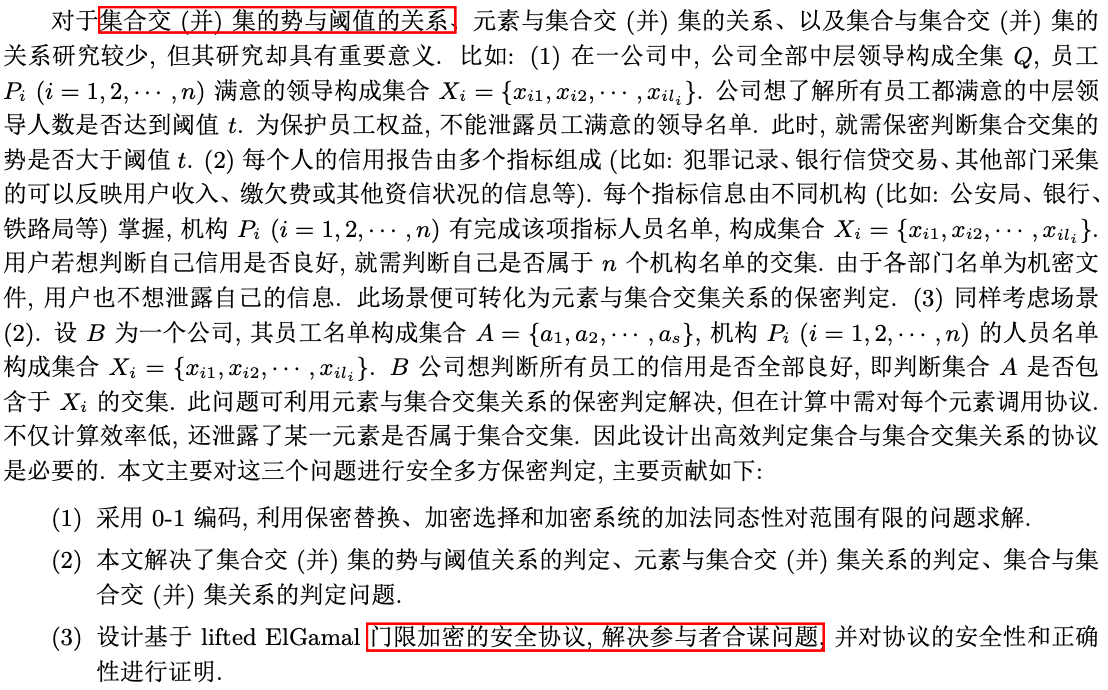（1）全部领导集合Q，全部员工集合P，员工满意领导的集合X，现想确定X中的数量大于阈值t
（2）用户信用判断（多个指标），每个机构P（一个指标）都有一个信用合格的用户名单X，现想确认一个用户是否在所有名单中X，即求一个元素是否在交集中。
（3）所有员工名单A，每个机构P（一个用户的信用指标）都有一个信用合格的用户名单X，现想判断A是否都在X的交集中，即一个集合是否在交集中。

## 预备知识

### 安全性定义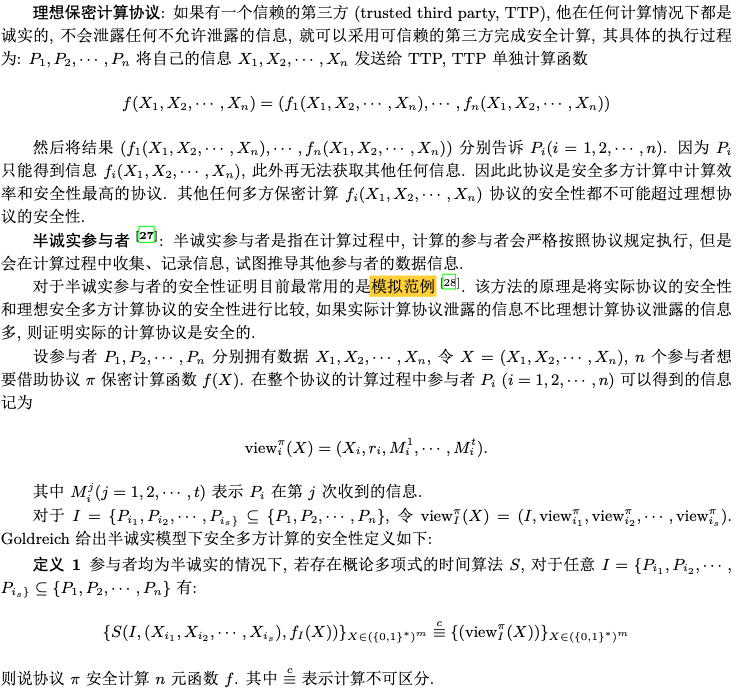### 门限密码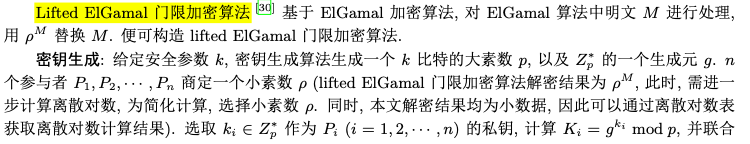（1）密钥生成和ElGamal类似，n个私钥$$k_i$$（每人一个），一个公钥$$h$$
（2）加密是和ElGamal一样的，密文是两部分
（3）解密，是需要所有人的私钥才能解密成功
（4）具有“加法同态性”：$$E(m_1)E(m_2)=E(m_1+m_2)$$，其实是密文相乘解密后相当于明文相加，当然这也算是具有同态性。
（5）这里的小素数$\rho$就是门限，有没有想到离散对数问题。

### 密文重随机化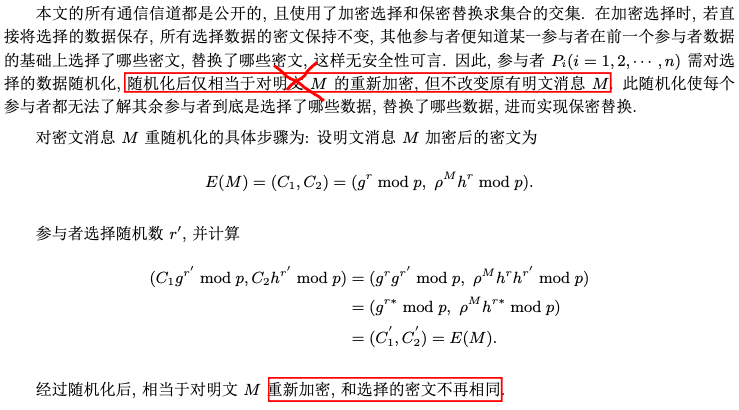## 集合的势与阈值的大小关系

### 交集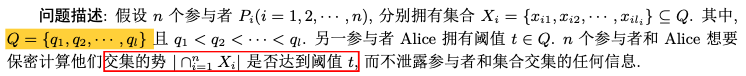#### 原理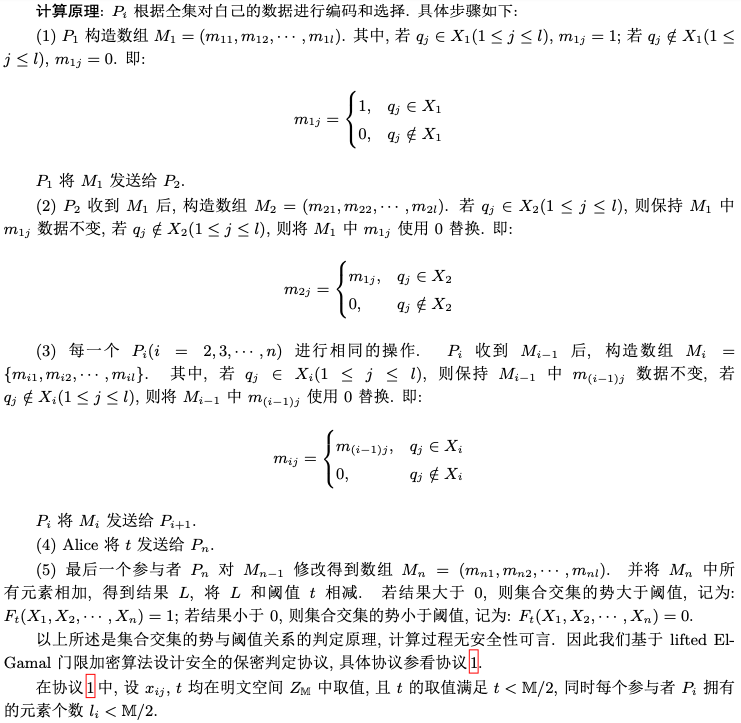• 这里的阈值$$t\in Q$$$$t$$$$Q$$按说是没啥关系吧？$$t$$就是一个数。
• 还有这里的$$Q$$$$l$$个元素，每个集合$$X$$也有$$l$$个元素，这个怎么搞？

Q=(1,2,3,4,5)
P1：X1=(1,2,3)
P2：X2=(2,3,4)
P3：X3=(3,4,5)
Alice：t=2

L=0+0+1+0+0=1
L-t<0，即输出为0

#### 方案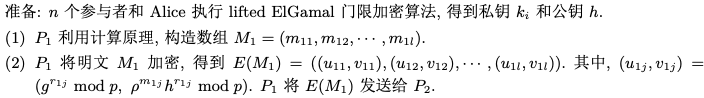（1）Alice将$$-t$$加密$$E(-t)$$,发送给$$P_n$$
（2）$$P_n$$$$E(M_n)$$中的元素逐个相乘得到$$E(L)$$，然后再将$$E(L)$$$$E(-t)$$相乘得到$$E(F)$$，最后将$$E(F)$$随机化，得到$$(u,v)$$，并公布$$u$$
（3）Alice和n个用户都是参与者，所以有$$n+1$$个私钥$$k_i$$
（4）Alice和(n-1)和用户各自计算$$t_i=u^{k_i}$$
（5）$$P_n$$解密，这里需要联合解密（n+1个参与者）
（6）注意这里$$E(-t)*E(L)=E(L-t)$$（别忘了加法同态性！）

### 并集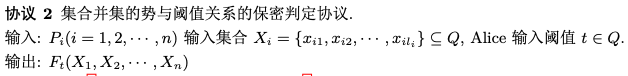#### 方案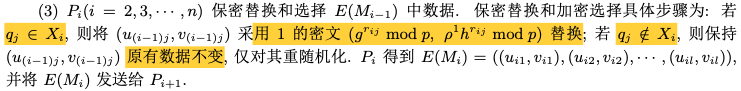（1）这里的“保密替换”和交集相反。

## 集合的交/并集与元素的包含关系

### 交集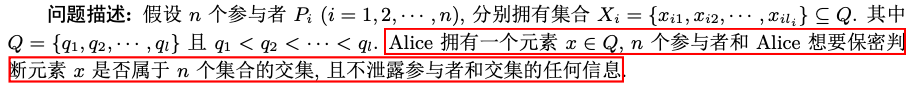#### 原理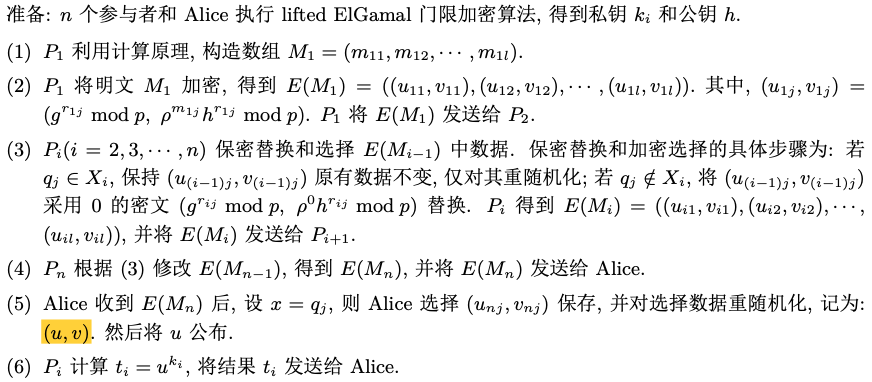#### 正确性和安全性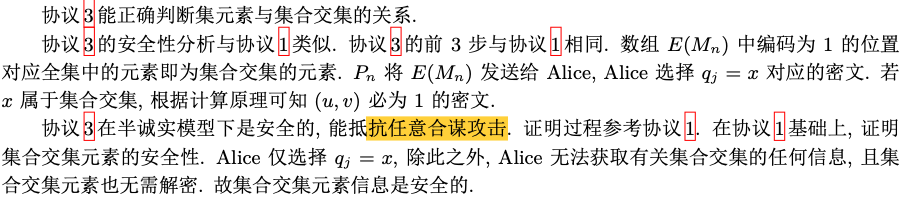### 并集#### 方案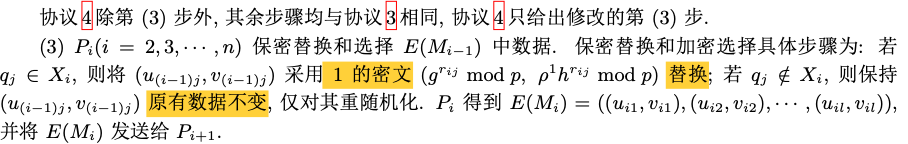## 集合的交/并集的判定

### 交集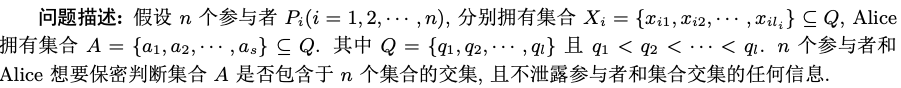#### 原理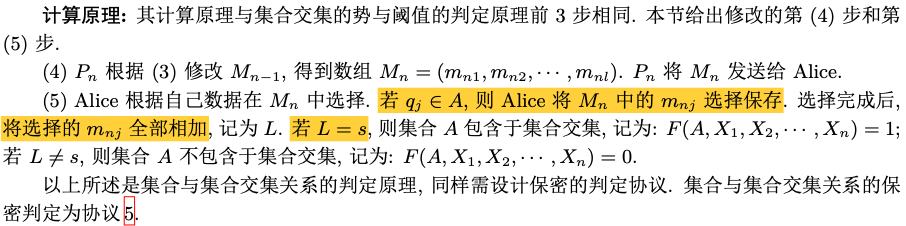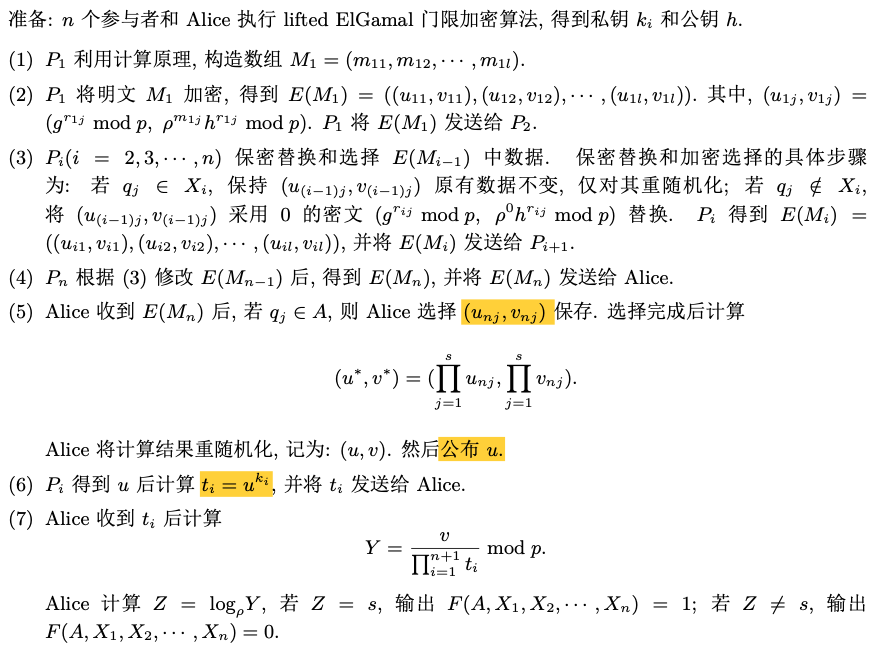（1）这里是Alice解密，$$P_i$$联合计算出$$t_i$$（n个）

#### 正确性和安全性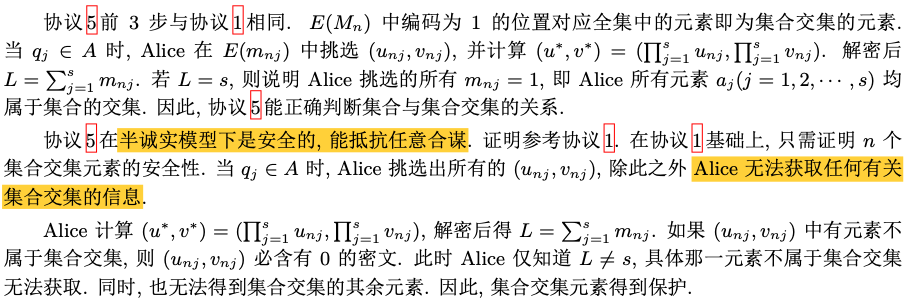### 并集#### 原理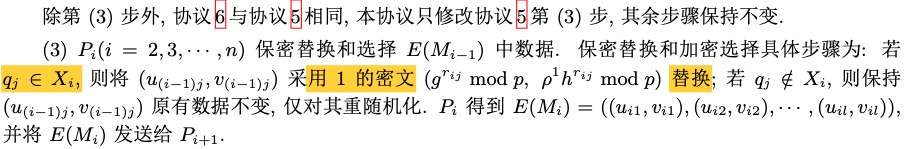## 性能

### 计算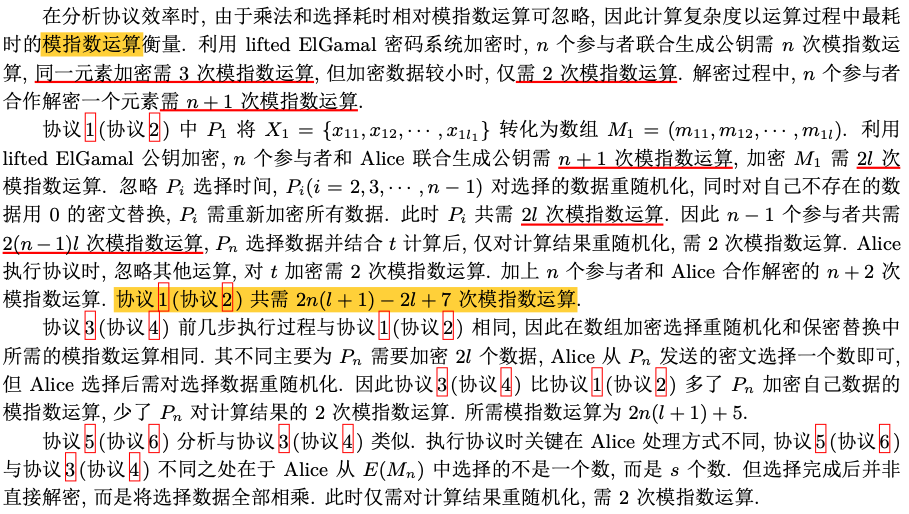### 通信## 总结### 低价透明### 金牌服务### 信息保密